# Generating missing values

To generate missing values in a dataset with missMethods you can use one of the delete_ functions. The names of these functions always starts with delete_ and the next part of the name shows the used missing data mechanism. There are three basic types of missing data mechanisms: missing completely at random (MCAR), missing at random (MAR) and missing not at random (MNAR). A list of all available functions for the different mechanisms is given below:

MCAR

• delete_MCAR()

MAR

• delete_MAR_1_to_x()
• delete_MAR_censoring()
• delete_MAR_one_group()
• delete_MAR_rank()

MNAR

• delete_MNAR_1_to_x()
• delete_MNAR_censoring()
• delete_MNAR_one_group()
• delete_MNAR_rank()

All these functions share a common interface. The first argument ds takes the dataset in which missing values should be generated. The next argument p specifies the proportion of missing values to include in every column with missing value. These columns are specified with the third argument cols_mis. The further arguments depend on the chosen function and are documented for every function separately. In most cases, reasonable defaults are set for these further arguments. Only the MAR functions need one additional argument with no default: cols_ctrl. The argument cols_ctrl specifies the columns that control the generation of missing data in a MAR settings.

One further remark: All MAR functions have a MNAR twin. These twins behave exactly the same way. The only difference is the columns that controls the generation of missing values. In the MAR functions separate cols_ctrl columns controls the generation of missing values in the cols_mis columns. In contrast, in the MNAR functions the generation of missing values in the cols_mis columns is controlled by the cols_mis columns themselves.

In the following, different examples for the generation of missing values will be presented. Furthermore, connections between the functions from missMethods and the paper by Santos et al. (2019) will be shown.

## Examples for the generation of missing values

The examples below show the use of some delete_ functions in a 2-dimensional dataset. Missing values are always generated in the variable “X” and 30 % of the values are deleted. At first, a basic set-up:

library(missMethods)
library(ggplot2)

set.seed(123)

make_simple_MDplot <- function(ds_comp, ds_mis) {
ds_comp$missX <- is.na(ds_mis$X)
ggplot(ds_comp, aes(x = X, y = Y, col = missX)) +
geom_point()
}

# generate complete data frame
ds_comp <- data.frame(X = rnorm(100), Y = rnorm(100))

### MCAR

Generate MCAR values:

ds_mcar <- delete_MCAR(ds_comp, 0.3, "X")
make_simple_MDplot(ds_comp, ds_mcar)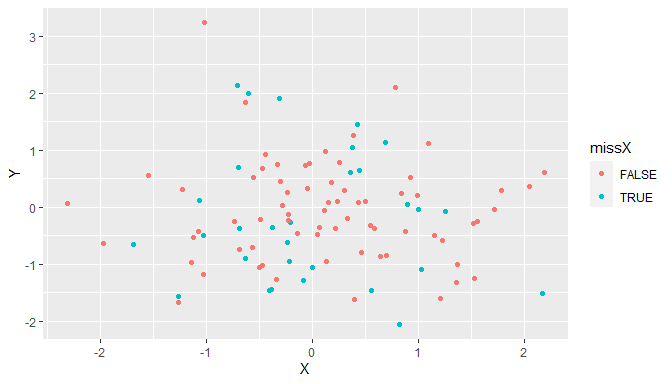### MAR

Generate MAR values using a censoring mechanism. This leads to a missing value in “X”, if the y-value is below the 30 % quantile of “Y”:

ds_mar <- delete_MAR_censoring(ds_comp, 0.3, "X", cols_ctrl = "Y")
make_simple_MDplot(ds_comp, ds_mar)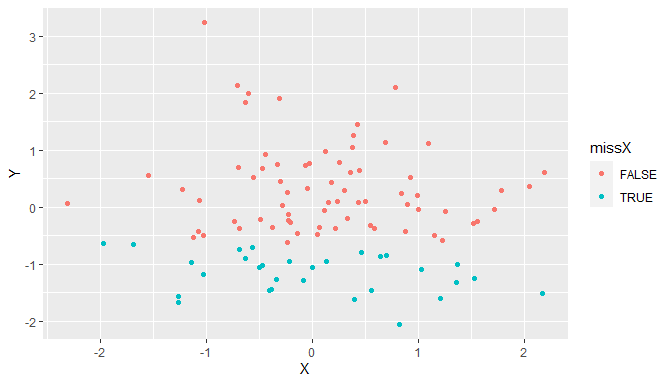The censoring mechanism is a rather strong form of MAR. A function that allows to control the strength of the MAR mechanism is delete_MAR_1_to_x. The strength is controlled through the argument x: the bigger x, the stronger the simulated MAR mechanism:

# x = 2
ds_mar <- delete_MAR_1_to_x(ds_comp, 0.3, "X", cols_ctrl = "Y", x = 2)
make_simple_MDplot(ds_comp, ds_mar)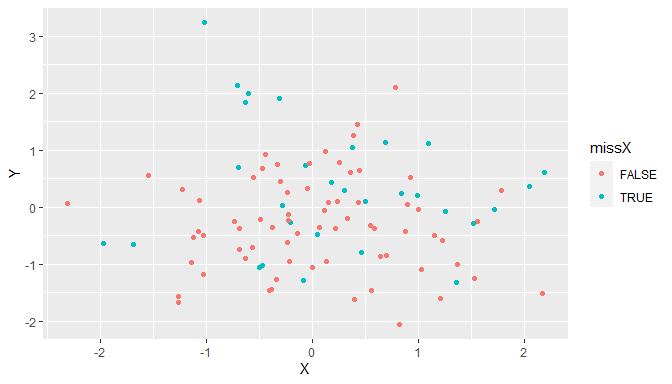# x = 10
ds_mar <- delete_MAR_1_to_x(ds_comp, 0.3, "X", cols_ctrl = "Y", x = 10)
make_simple_MDplot(ds_comp, ds_mar)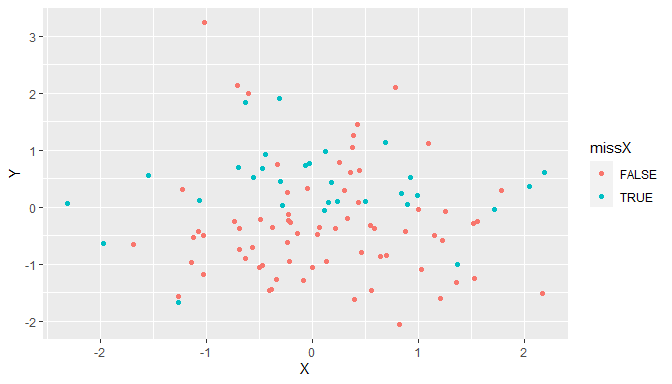### MNAR

Generate MAR values using a censoring mechanism. This leads to a missing value in “X”, if the x-value is below the 30 % quantile of “X”:

ds_mnar <- delete_MNAR_censoring(ds_comp, 0.3, "X")
make_simple_MDplot(ds_comp, ds_mnar)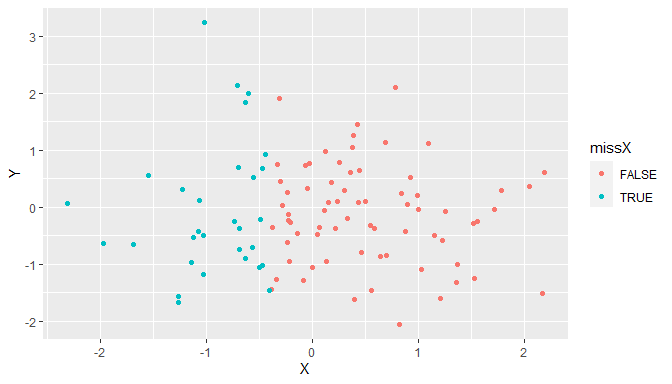## Connections between missMethods and Santos et al. (2019)

The following table shows the connections between the algorithm names of the missing data creation methods in Santos et al. (2019) and the functions of missMethods:

Santos et al. (2019) Function Arguments
MCAR1univa delete_MCAR stochastic = FALSE
MCAR2univa delete_MCAR all default
MCAR3univa delete_MCAR all default
MAR1univa delete_MAR_censoring sorting = FALSE
MAR2univa delete_MAR_rank all default
MAR3univa delete_MAR_1_to_x x = 1/9
MAR4univa delete_MAR_censoring where = “upper”
MAR5univa delete_MAR_censoring where = “both”
MNAR1univa delete_MNAR_censoring sorting = FALSE
MNAR2univa delete_MNAR_censoring where = “upper”
MCAR1unifo delete_MCAR stochastic = FALSE
MCAR2unifo delete_MCAR p_overall = TRUE
MAR1unifo delete_MAR_censoring all default
MAR2unifo delete_MAR_censoring sorting = FALSE
MAR3unifo delete_MAR_one_group all default
MAR4unifo delete_MAR_one_group all default
MNAR1unifo delete_MNAR_censoring all default
MNAR2unifo delete_MNAR_censoring sorting = FALSE
MNAR3unifo delete_MAR_one_group all default
MNAR4unifo delete_MAR_one_group all default

Only the argument(s), which default values must be altered, are shown in the table. Notice that most functions in missMethods are more general than the described algorithms in Santos et al. (2019). Therefore, some functions of missMethods are able to replace different algorithms from Santos et al. (2019). In contrast to Santos et al. (2019), the user must always specify the missing column(s). However, this may change in the future.